# Excel VBA Break a Loop with Keyboard Shortcut

Get FREE Advanced Excel Exercises with Solutions!

In this article, we will show how to break a VBA loop with keyboard keys in Excel. Excel users often encounter infinite loops that run for an infinite time. These loops are harmful to the system in which they are running. Also, if a VBA code is applied to a large sample of data, the code struggles to execute and often ends up being slow or harming the system. An immediate exit is the only way to resolve the issue. Here, we will learn about different keyboard shortcuts to exit the loop prematurely.

## How to Open VBA Macro Editor in Excel

In order to run any VBA code, we first need to write or edit the code in the VBA Macro Editor.

Before that, we need to enable the Developer tab in our Excel sheet. The Developer tab allows users to access the VBA Macro Editor.

Follow the simple steps below to open up the VBA Macro Editor.• Go to Developer Tab >> Visual Basic.
• This will open the Visual Basic window.• Select Insert >> Module in the macro editor.• As a result, an empty module will appear on the screen where you can write the code.

## How to Break VBA Loop with Keyboard Keys in Excel: 5 Cases

Here, we have a dataset of the marks of some students in three subjects and the average marks. The function of the VBA codes is to assign a grade to each student based on their average marks. In this section, we will show 5 cases where we need to use the keyboard keys to break a VBA loop. We will show four cases of the most widely used loops in VBA, namely: the For Loop, While Loop, and Do While Loop. In each case, the loops will run indefinitely due to the wrong assignment of variables. In the last case, we will show the execution of the For Loop on a large dataset and how to break away from that code using keyboard shortcuts Ctrl+Break and Ctrl+Fn+Break.

### Case 1: Breaking Infinite For Loop Using Keyboard Shortcuts

In the image below, we see a VBA code with a For Loop. Due to the reassignment of i variable, the code will run in an infinite loop. Thus, it becomes important to break out of the code. We will use the Ctrl+Break and Ctrl+Fn+Break keyboard shortcuts to do so.``````Sub ForLoop()
Dim i As Integer
For i = 5 To 10
Select Case Cells(i, 6).Value
Case 60 To 69: Cells(i, 7).Value = "D"
Case 70 To 79: Cells(i, 7).Value = "C"
Case 80 To 89: Cells(i, 7).Value = "B"
Case 90 To 100: Cells(i, 7).Value = "A"
Case Else: Cells(i, 7).Value = "Failed"
End Select
i = 5
Next i
End Sub``````

VBA Breakdown

``````Sub ForLoop()
Dim i As Integer``````
• Here, we defined a sub-procedure ForLoop to execute the operation. Then, we took an Integer variable i.
``````For i = 5 To 10
Select Case Cells(i, 6).Value
Case 60 To 69: Cells(i, 7).Value = "D"
Case 70 To 79: Cells(i, 7).Value = "C"
Case 80 To 89: Cells(i, 7).Value = "B"
Case 90 To 100: Cells(i, 7).Value = "A"
Case Else: Cells(i, 7).Value = "Failed"
End Select
i = 5
Next i``````
• Next, we initiated a for loop with conditional statements inside it. Inside the For Loop, we ran the loop six times, as the variable holds all the integers between 5 and 10, inclusive. Then we set a conditional statement. For each value of i, the code will look at the cell values in the F column, starting from row 5 up to row 10. Depending on the value of the cell, it will assign a grade to the adjacent cell, which is in the G column. Here, we have 5 cases and five grades. The code will assign a D grade if the value in the F column is between 60 and 69. Similarly, it will continue to assign grades in the G column according to the rest of the conditions. However, at the end of the condition statement, we set the value of i to 5 again. So, at the end of each loop, the value of i variable becomes 5. This makes the loop an infinite loop. Hence, we will use the keyboard buttons to terminate it.
• In the 1.1 and 1.2 sections below, we display two different ways to break the For Loop using keyboard shortcuts.

####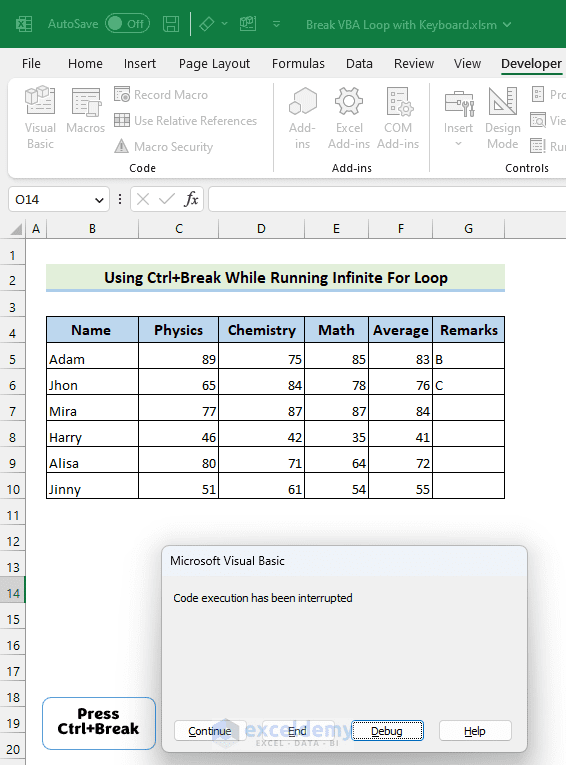As the overview video suggests, first we will run the code by going to Developer >> Macros. Then, we will select the ForLoop sub-procedure. The code will run accordingly. However, in order to break out of the loop, we will press the Ctrl+Break buttons on the keyboard. Excel will show “Code execution has been interrupted.” Finally, we will press End to finish the termination process.

####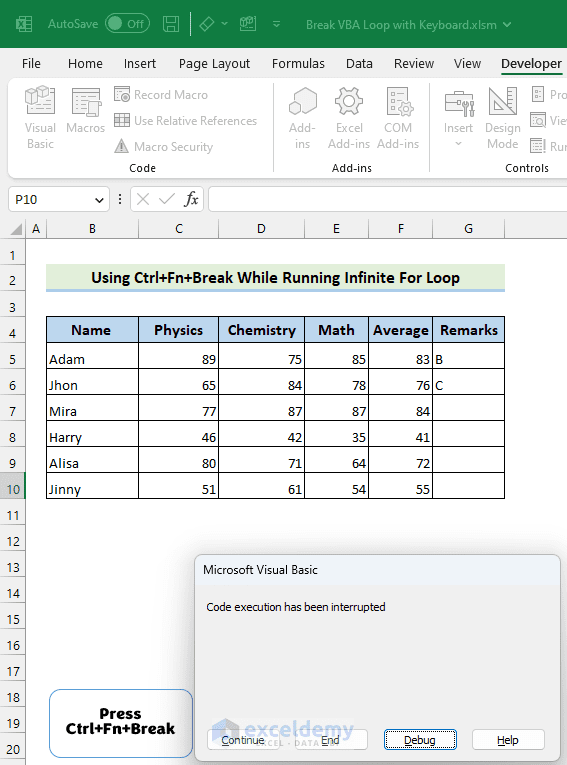Just like the video above, first, we will execute the code by selecting Developer >> Macros. Secondly, we will choose the ForLoop sub-procedure. The program will execute as planned. However, we will press the Ctrl+Fn+Break keyboard shortcuts to exit the loop. As a result, Excel will show an error message in a prompt. We’ll finally click End to complete the termination process.

### Case 2: Break Out of Infinite While Wend Loop Applying Keyboard Keys

In the ensuing picture, we see a While Loop. Here, the assignment of the variable i becomes such that the condition never gets satisfied, ultimately leading to the creation of an infinite While Loop which we will break with keyboard keys.``````Sub WhileLoop()
Dim i As Integer
i = 5
While i < 6
Select Case Cells(i, 6).Value
Case 60 To 69: Cells(i, 7).Value = "D"
Case 70 To 79: Cells(i, 7).Value = "C"
Case 80 To 89: Cells(i, 7).Value = "B"
Case 90 To 100: Cells(i, 7).Value = "A"
Case Else: Cells(i, 7).Value = "Failed"
End Select
i = 5
Wend
End Sub``````

VBA Breakdown

``````Sub WhileLoop()
Dim i As Integer
i = 5``````
• Here, we created a sub-procedure called While Loop to carry out the action. We also took an integer variable named i. We then initiated the value of i variable 5.
``````While i < 6
Select Case Cells(i, 6).Value
Case 60 To 69: Cells(i, 7).Value = "D"
Case 70 To 79: Cells(i, 7).Value = "C"
Case 80 To 89: Cells(i, 7).Value = "B"
Case 90 To 100: Cells(i, 7).Value = "A"
Case Else: Cells(i, 7).Value = "Failed"
End Select
i = 5
Wend``````
• The While Loop will continue to run until the value of i becomes greater than 6. Inside the loop, the conditional statements are the same as the For Loop mentioned in the previous case. Here too, the value of i will become 5 at the end of each loop. So, it will never be greater than 6. So the loop will run infinitely.
• In sections 2.1 and 2.2, we show the output of breaking the While Loop using keyboard shortcuts.

#### 2.1 Using Ctrl+Break/Pause KeysSame as the examples before, here too, first we will run the code by going to Developer >> Macros. Then, we will select the WhileLoop sub-procedure. The code will run accordingly. However, in order to break out of the loop, we will press the Ctrl+Break buttons on the keyboard. Excel will show “Code execution has been interrupted.” Finally, we will press End to finish the termination process.

#### 2.2 Applying Ctrl+Fn+Break KeysAs indicated in the overview video, in this instance, we will execute the code first by selecting Developer >> Macros. After that, we will choose the WhileLoop sub-procedure. The program will execute as planned. However, we will press the Ctrl+Fn+Break keyboard shortcuts to exit the loop. As a result, Excel will show an error message in a prompt. We’ll finally click End to complete the termination process.

Read More: Excel VBA Break While Loop

### Case 3: Breaking Infinite Do While Loop with Keyboard Shortcut

In this instance, we will break out of an infinite Do While Loop by using Ctrl+Break and Ctrl+Fn+Break. The code that will result in an infinite loop due to the assignment of i variable is as follows.``````Sub DoWhileLoop()
Dim i As Integer
i = 5
Do While i < 6
Select Case Cells(i, 6).Value
Case 60 To 69: Cells(i, 7).Value = "D"
Case 70 To 79: Cells(i, 7).Value = "C"
Case 80 To 89: Cells(i, 7).Value = "B"
Case 90 To 100: Cells(i, 7).Value = "A"
Case Else: Cells(i, 7).Value = "Failed"
End Select
i = 5
Loop
End Sub``````

VBA Breakdown

• Like the WhileLoop sub procedure, here too we set the value of an integer variable i to 5. However, the value of the variable will be reassigned to 5 after each loop and the condition at the beginning of the Do While will never be satisfied, and thus it will run infinitely.
• In the following two sections (sections 3.1 and 3.2) we display two ways to break the Do While Loop.

####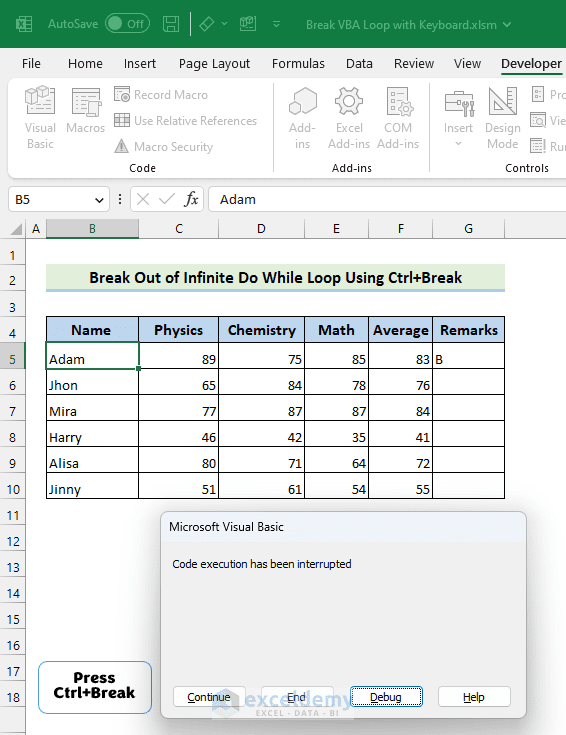In this case, we will run the code first by selecting Developer >> Macros. Then, we will choose the DoWhileLoop sub-procedure. The program will execute as planned. Next, we will press the Ctrl+Break keyboard shortcuts to exit the loop. Consequently, Excel will show an error message in a prompt. We’ll finally click End to complete the termination process.

#### 3.2 Utilizing Ctrl+Fn+Break KeysHere, we will initiate the code first by selecting Developer >> Macros. After that, we will select the DoWhileLoop sub-procedure. The program will start to execute accordingly. However, we will press the Ctrl+Fn+Break keyboard shortcuts to exit the loop. As a result, Excel will show an error message in a prompt. We’ll finally click End to complete the termination process.

### Case 4: Break Infinite Loop While Running VBA in Message Box

As the video suggests, we will run the following code with a While Loop whose condition will never be satisfied because of the reassignment of i variable. So, the output of MsgBox will run indefinitely. We will break this loop by applying keyboard shortcuts.``````Sub LoopInMsgBox()
Dim i As Integer
i = 5
While i < 6
Select Case Cells(i, 6).Value
Case 60 To 69: MsgBox "D"
Case 70 To 79: MsgBox "C"
Case 80 To 89: MsgBox "B"
Case 90 To 100: MsgBox "A"
Case Else: MsgBox "Failed"
End Select
i = 5
Wend
End Sub``````

VBA Breakdown

• In this case, we first declared a sub-procedure called LoopInMsgBox. We set the variable, While  Loop condition, and the conditional statements the same as the WhileLoop sub procedure. However, the only difference is, that here, we will show the grades of the students in  MsgBoxes other than in a cell.
• In sections 4.1 and 4.2, we display two output images that show two different ways to break out of an infinite loop.

####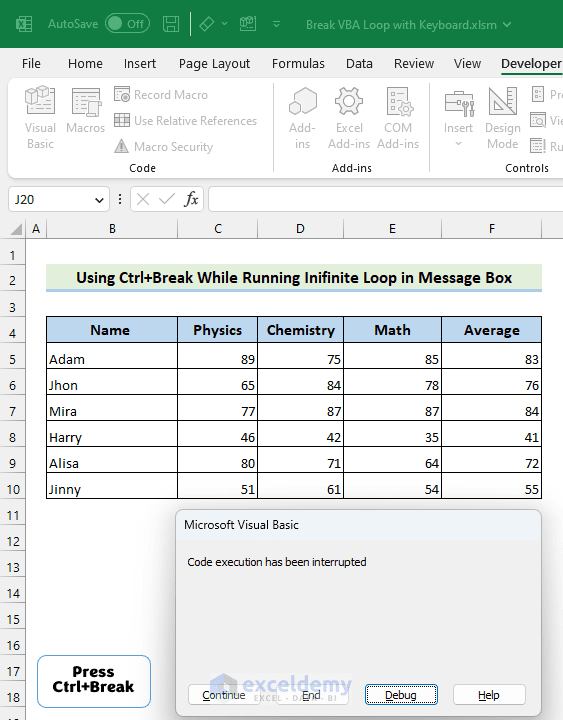In this instance, we’ll choose Developer >> Macros and run the code first. We will next select the LoopInMsgBox sub-procedure. Program execution will go as expected. To break the loop, we will use the keyboard commands Ctrl+Break. Excel will consequently display an error message in a prompt. Finally, we’ll select End to finish the termination procedure.

####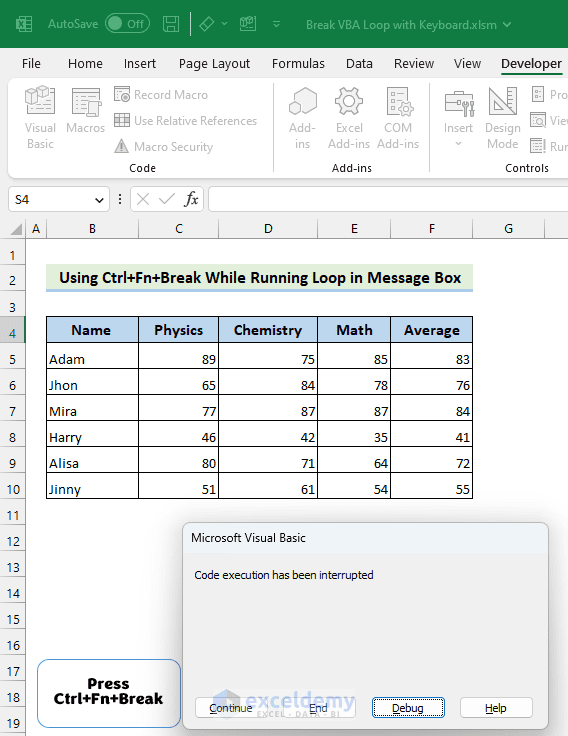First, we will run the code by choosing Developer >> Macros. Then, we will select the LoopInMsgBox sub-procedure. Program execution will go as expected. To break the loop, we will instead press the keyboard commands Ctrl+Fn+Break. Excel will display an error message as a prompt. Finally, we’ll select End to finish the termination procedure.

### Case 5: Breaking Loop Using Keyboard Shortcut for Large Dataset

Here, we take a dataset with 1000 data. We will run a For Loop on this dataset. Due to this large dataset, the code will take time to complete the execution. In order to break out of this code and save time, we will use a set of keyboard keys.``````Sub LargeDataset()
Dim i As Integer
For i = 5 To 1004
Select Case Cells(i, 6).Value
Case 60 To 69: Cells(i, 7).Value = "D"
Case 70 To 79: Cells(i, 7).Value = "C"
Case 80 To 89: Cells(i, 7).Value = "B"
Case 90 To 100: Cells(i, 7).Value = "A"
Case Else: Cells(i, 7).Value = "Failed"
End Select
Next i
End Sub``````

VBA Breakdown

• Here, we created a sub-procedure named LargeDataset. Then, we took an integer variable i. We change the value of the variable i from 5 to 1004 and run the for loop 1000 times. At each iteration, the code assigns a grade to the cells in the G columns. This is a highly repetitive task as the dataset is large. Hence, the code will take time to execute.

Here, the dataset consists of 1000 data. The For Loop will take a good amount of time to go over all the iterations. So, one can press the Ctrl+Break or Ctrl+Fn+Break keys to break out of the loop. I Hope this method gave you an idea to break loop with keyboard shortcuts in Excel VBA.

## How to Disable Debugging in Excel

We often share our Excel file with VBA codes in it. However, the code that we write can be debugged by another person. If we want to keep our code intact, we need to disable debugging. Follow the steps below to do so-• Choose Developer Tab >> Visual Basic.• From the Visual Basic Window, select Tools >> VBAProject Properties.
• As a result, a prompt will be opened.• In the prompt, first, go to the Protection tab.
• Mark “Lock project for viewing”.
• Insert a password and then confirm it.
• Finally, click on OK.

## What Is Run Time Error 1004 in Excel Macro?

The Run Time Error 1004 is a very common type of error in Excel. When the macro code tries to execute an operation on an object or range, but the object is either not present or not reachable, Runtime Error 1004 in Excel macros occurs.Here, in this video, as we tried to execute the RunTimeError sub-procedure, Excel showed a run time error. So, we debugged the code. As a result, we find that we set a Range object Rng which starts from Alpha1. But there is no cell called Alpha1 in that worksheet. So, the Rng object did not exist. This is why Excel showed the run time error.So, we fixed the code and replaced Alpha1 with A1 which is a valid cell in the worksheet

Now, if we run the code, there will be no run time error.

• What is a Breakpoint in VBA?

In VBA (Visual Basic for Applications), a breakpoint is a tool that enables you to stop the execution of your code at a particular line in order to inspect the state of your program at that time. When you are debugging your code, this is very helpful since it enables you to identify the precise position of a problem and look at the values of variables and other data structures.

• How can I avoid getting stuck in an infinite loop while running my Excel VBA code with the keyboard?

Getting stuck in an infinite loop is a common issue while running Excel VBA code, and it can be frustrating. Here are a few ways to resolve the issue:

• Use Breakpoints at key locations of your code. This will help you to figure out the reason behind the creation of the infinite loop.
• Add error handling to your code to identify any errors that may occur and prevent your code from getting stuck in a loop.
• Use the “Step Into” or “Step Over” options in the VBA editor to execute your code one line at a time. This will help you figure out any issues that may cause your code to get stuck in a loop.

## Things to Remember

• If the keyboard of your computer does not have any of the keys( Ctrl, Break,Fn) then do not worry. Just press Ctrl+Windows+O button to open up the on-screen keyboard. Then, you will have the required keys to terminate the program.
• If you are using Mac then
• Press Command + Spacebar to open Spotlight Search.
• Type “Keyboard Viewer” in the search bar.
• Press Enter.

This will open the on-screen keyboard on your Mac. Alternatively, you can also access the on-screen keyboard by going to the “Input Sources” menu in the top-right corner of your screen, clicking on “Show Keyboard Viewer,” and the on-screen keyboard will appear. This will provide you with the required keys to break the loops.

• We can terminate the entire Excel application by pressing Ctrl+Alt+Delete to open up the Task Manager in Windows and then clicking on the Excel application followed by pressing End Task. This will not only terminate the loop but also the entire Excel program.

## Conclusion

In this article, we have seen 5 cases where we used keyboard shortcuts to break an Excel VBA loop. These shortcuts will allow users to break infinite loops immediately, which are potentially dangerous for the operating system where they are running.

## What is ExcelDemy?

ExcelDemy Learn Excel & Excel Solutions Center provides free Excel tutorials, free support , online Excel training and Excel consultancy services for Excel professionals and businesses. Feel free to contact us with your Excel problems.I am an engineering graduate. I graduated from Bangladesh University of Engineering and Technology(BUET), one of the top universities in Bangladesh from department of Naval Architecture & Marine Engineering with a major in structure. I am an avid reader of fiction especially fantasy. I also keep myself abreast of the recent developments in science and technology. I believe diligence will eventually pay off and luck tends to favor those who work hard.

We will be happy to hear your thoughtsAdvanced Excel Exercises with Solutions PDF